Next: Support vector machines and Up: Regression Previous: Exact predictive density   Contents

Gaussian mixture regression (cluster regression)

Generalizing Gaussian regression the likelihoods may be modeled by a mixture of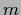Gaussians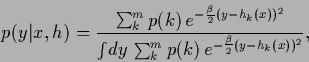(331)

where the normalization factor is found as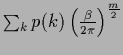. Hence,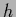is here specified by mixing coefficients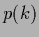and a vector of regression functions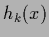specifying the-dependent location of the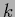th cluster centroid of the mixture model. A simple prior foris a smoothness prior diagonal in the cluster components. As any density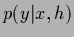can be approximated arbitrarily well by a mixture with large enoughsuch cluster regression models allows to interpolate between Gaussian regression and more flexible density estimation.

The posterior density becomes for independent data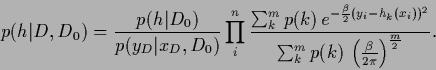(332)

Maximizing that posterior is -- for fixed, uniformand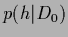-- equivalent to the clustering approach of Rose, Gurewitz, and Fox for squared distance costs .Next: Support vector machines and Up: Regression Previous: Exact predictive density   Contents
Joerg_Lemm 2001-01-21